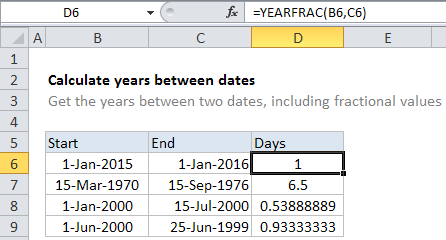## Excel Office

Excel How Tos, Tutorials, Tips & Tricks, Shortcuts

# Calculate years between dates in Excel

If you want to calculated the number of years between two dates,  then this tutorials is for you. See example below;

To calculated the number of years between two dates, you can use the YEARFRAC function, which will return a decimal number representing the fraction of a year between two dates. Here are a few examples of the results that YEARFRAC calculates:

Worked Example:   Use of INT and TRUNC function in Excel

## Formula

`=YEARFRAC(start_date,end_date)`## Explanation

Note: The YEARFRAC function has an optional 3rd argument that controls how days are counted when computing fractional years. The default behavior is to count days between two dates based on a 360-day year, where all 12 months are considered to have 30 days. The YEARFRAC page provides more information.

Worked Example:   Round numbers in Excel
 Start date End date YEARFRAC result 1/1/2015 1/1/2016 1 3/15/1970 9/15/1976 6.5 1/1/2000 7/15/2000 .5389 6/1/2000 6/25/1999 .9333

Once you have the decimal value, you can round the number if you like. For example, you could round to the nearest whole number:

`=ROUND(YEARFRAC(A1,B1),0)`

You might also want to keep only the integer portion of the result with no fractional value, so that you are only counting whole years. In that case, you can just wrap YEARFRAC in the INT function:

`=INT(YEARFRAC(A1,B1))`